# Bracing with ribs

## 1. Introduction

The ribs have stiffening effect. So the plates are supplied with different ribs.

## 2. Running the case

The command line to run this case is

``mpirun -np 4 feelpp_toolbox_solid --case "github:{repo:toolbox,path:examples/modules/csm/examples/ribs}"``

## 3. Data files

The case data files are available in Github here

## 4. Model/Geometry

The first step is to create the model of the plate, which we can simply do in the Creo Parametric program. With this program was the fastest and easiest way to create the model. The finished geometry (Creo) and the meshed model (Gmsh):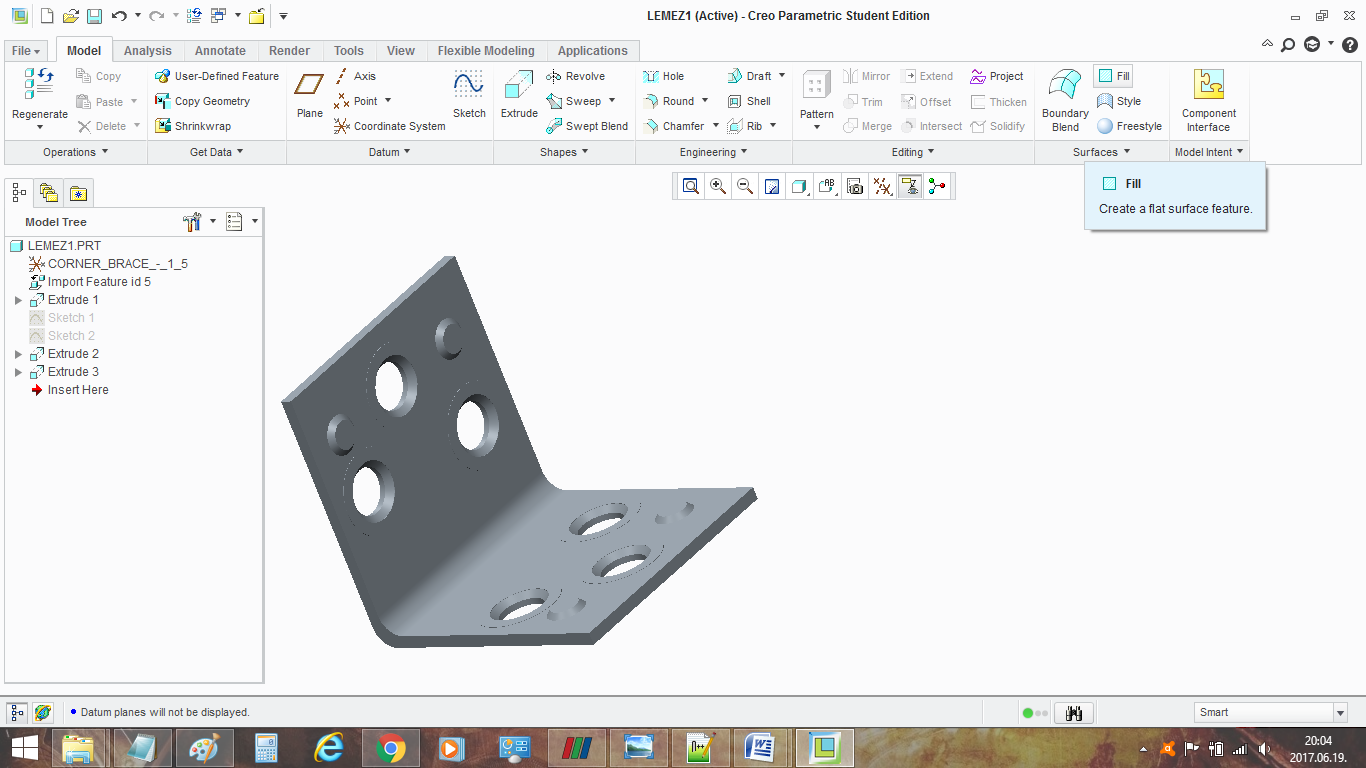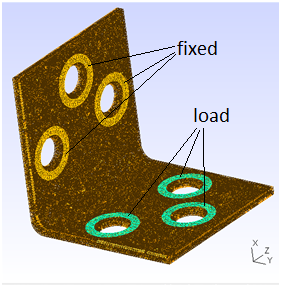## 5. Materials and boundary conditions

### 5.1. Materials

The beam is made of steel, so we need to use the following data:

• E = 210 GPa → 210e3 N/mm2

• nu = 0.3

• rho = 7800 kg/m3 → 7800e-9 kg/mm3

``````    "Materials":
{
"omega":
{
"E":"210e3",		// N/mm^2
"nu":"0.4",			//
"rho":"7800e-9"		// kg/mm^3
}
},``````

### 5.2. Boundary conditions

The plate is fixed with three screws (yellow → named `fixed`). The force is applied, where are the other three srews (green → named `load`).

``````    "BoundaryConditions":
{
"displacement":
{
"Dirichlet":
{
"fixed":
{
"expr":"{0,0,0}"
}
},
"Neumann_scalar":
{
{
"expr":"1e0"
}
}
}
},``````

## 6. Results (Displacement and Von-Mises Criterions)

### 6.1. Without ribs

Maximum displacement: 0.6501 mm Maximum stress: 512.4 MPa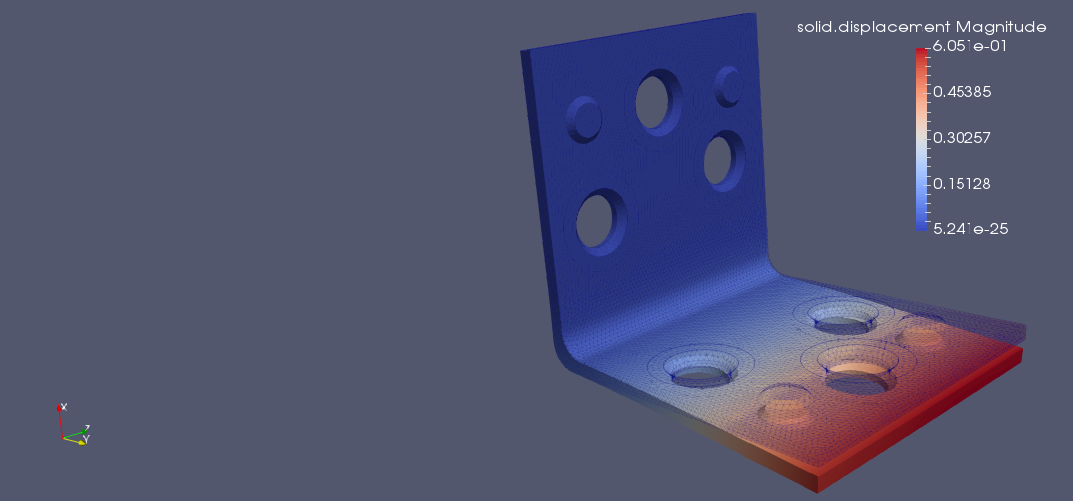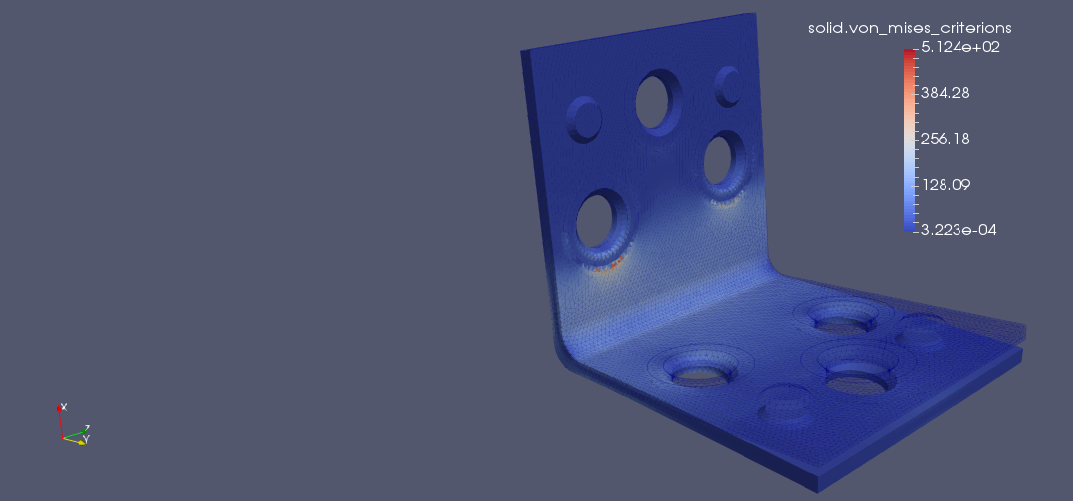### 6.2. With ribs

Maximum displacement: 0.528 mm Maximum stress: 599.1 MPa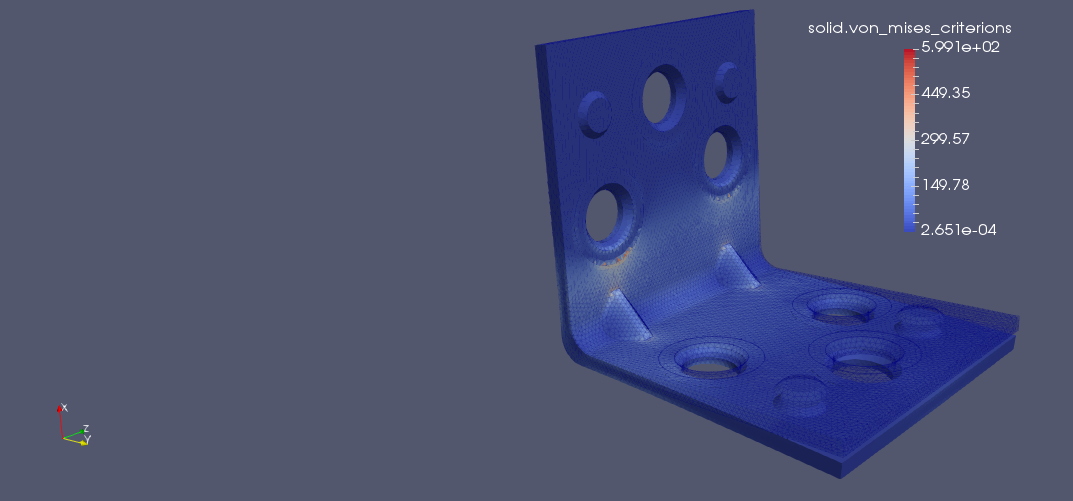### 6.3. Summary

The deformation of the plate is smaller (-18%) with ribs.

The maximum stress bigger, but in the bend where the plate would break, there is smaller.